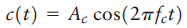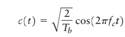Create an Account

Home / Questions / Throughout this chapter we have expressed the sinusoidal carrier wave in the form where ...

Throughout this chapter we have expressed the sinusoidal carrier wave in the form where Ac is the carrier amplitude and is the carrier frequency In Chapter 7 dealing with digital band pass

Throughout this chapter, we have expressed the sinusoidal carrier wave in the formwhere Ac is the carrier amplitude and is the carrier frequency. In Chapter 7 dealing with digital band-pass modulation techniques, we find it more convenient to express the carrier in the formwhere Tb is the duration allotted to the transmission of symbol 1 or symbol 0. Determine the value of carrier amplitude Ac for the energy in per symbol to equal unity.

Jul 31 2020 View more View LessSubscribe To Get Solution